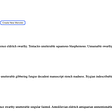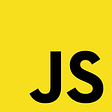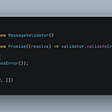# Introduction to Monads in Javascript (Video)

The idea : When we use map on a function f: a→ b we transform the value A inside the functor T(A) (using the container metaphor ) to a new type T(b) — Sometimes we may happen that type b is itself a T(a) so the function that we want to map is something like that f: a→T(a) In those situations we end up with something like T(T(a)) a Box in a Box situation (a Functor in a Functor) if T is a Monad it has a method Join that allows us to go back to the initial state T(a) or “unwrap” one layer.

For example, for the Identity Functor

`const Id = (v) => ({  value: v,  map: (f) => Id(f(v))});`

if we use a function inside the map that returns an Id

`Id(5).map(x=>Id(x+1))`

Then we end up with this `Id(Id(6))` how would you get out of this ? well if we drop the Id on the map `map: (f) => Id (f(v))` would be fine. As you can see at the next figure, now after applying the function f at step 2 we just return the result immediately

So just for those times when we want to map to a functor we use another method instead of map called bind `bind:(f) =>f(v)`,

`Id(5).bind(x=>Id(x+1))  == Id(6)`

The same reasoning goes for all functors, for example for the Array functor we can get the array-in-array situation easily:

`[5,6].map(x=>[x+1]) === [, ]`

The native array has a bind operation that is called flatMap [which actually is another usual name the Object-oriented Programming community likes to call the monadic bind]. So we should have used flatMap in the above example in order to get the correct array

`[5, 6].flatMap(x => [x + 1]) === [5, 6]`

This might seem confusing, but the bind is a combination of two functors in one.

We are going to see some of the Monads. The most important are the Maybe,Either,and Promise :

….

Excerpt from the Book Functional Programming in Javascript in LeanPub

Get the complete set of video lectures containig realistic examples of Maybe,Either and Promises totally free

initially published in https://www.fluidinfunctional.com/

--

--

--

More than 14 years’ experience as full stack developer and Software Architect .https://leanpub.com/u/dimitrispapadim

Love podcasts or audiobooks? Learn on the go with our new app.

## Generics in Typescript using Real time Example## How to create admin dashboard with charts using Reactjs and D3js## Getting the Monsters Under Control in a Single-Page Application## Content Script — Google Chrome Extension## Vue.js and AWS AppSync, part one## Web Socket Integration With Spring Boot.## JavaScript: Can (a==1 && a==2 && a==3) ever evaluate to true?## Trend — Vue, React & AngularMore than 14 years’ experience as full stack developer and Software Architect .https://leanpub.com/u/dimitrispapadim

## Logical Assignment Operators in JavaScript## Understanding arrow functions in JS## How Hoisting Works in JavaScript — Variables and Functions## Async function from callback in JavaScript